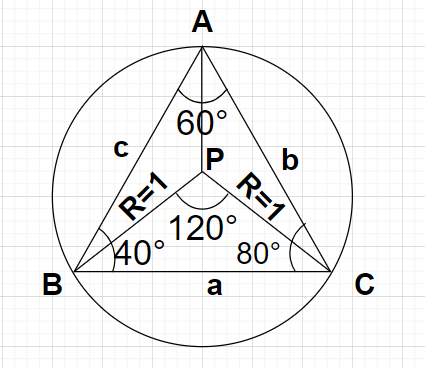Courses
Courses for Kids
Free study material
Free LIVE classes
MoreLIVE
Join Vedantu’s FREE Mastercalss

# In triangle ABC, $\angle {\text{A}} = {60^0},\angle {\text{B}} = {40^0}$ and $\angle {\text{C}} = {80^0}$. If P is the centre of the circumcircle of triangle ABC with circumradius unity, then what is the radius of circumcircle of triangle BPC.${\text{A}}{\text{. 1}} \\ {\text{B}}{\text{. }}\sqrt 3 \\ {\text{C}}{\text{. 2}} \\ {\text{D}}{\text{. }}\dfrac{{\sqrt 3 }}{2} \\$Verified
361.2k+ views
Hint- Here, we will be using the extended sine rule which gives the relation between the length of the sides of the triangle and the circumradius of that triangle i.e., $\dfrac{a}{{\sin {\text{A}}}} = \dfrac{b}{{\sin {\text{B}}}} = \dfrac{c}{{\sin {\text{C}}}} = 2{\text{R}}$.

Given a triangle ABC with $\angle {\text{A}} = {60^0},\angle {\text{B}} = {40^0}$ and $\angle {\text{C}} = {80^0}$ as shown in the figure.
Let the length of the side opposite to vertex A is a, that of the side opposite to vertex B is b and that of the side opposite to vertex C is c as shown in the figure.
Now, P is the centre of the circumcircle of triangle ABC whose circumradius is unity i.e., R=1
In any triangle ABC with sides AB, BC and AC of length a, b and c respectively and with R being the radius of the circumcircle of triangle ABC.
According to the extended sine rule, we can write
$\dfrac{a}{{\sin {\text{A}}}} = \dfrac{b}{{\sin {\text{B}}}} = \dfrac{c}{{\sin {\text{C}}}} = 2{\text{R }} \to (1)$
Using formula given by equation (1), we have
$\dfrac{a}{{\sin {\text{A}}}} = 2{\text{R}}$
By putting $\angle {\text{A}} = {60^0}$ and R=1, the above equation becomes
$\dfrac{a}{{\sin {{60}^0}}} = 2 \times 1 \\ \Rightarrow a = 2\left( {\sin {{60}^0}} \right) \\$
According to trigonometric table $\sin {60^0} = \dfrac{{\sqrt 3 }}{2}$ so the above equation becomes
$\Rightarrow a = 2\left( {\dfrac{{\sqrt 3 }}{2}} \right) = \sqrt 3$
So, the length of the side BC is $\sqrt 3$
According to the inscribed angle theorem, if $\theta$ is the inscribed angle in a circle by some arc then the central angle which the same arc subtends on the circle is equal to $2\theta$.
Using the inscribed angle theorem, we can write
$\angle {\text{BPC}} = 2\angle {\text{A}} \\ \Rightarrow \angle {\text{BPC}} = 2\left( {{{60}^0}} \right) = {120^0} \\$
Now, if we observe the triangle BPC. Here, the sides BP and PC are the circumradius of the circumcircle of the triangle ABC i.e., BP=PC=R=1. Also, the length of side BC is a.
Let the circumradius of the circumcircle of the triangle BPC is r.
According to extended sine rule for triangle BPC, we can write
$\dfrac{a}{{\sin \left( {\angle {\text{BPC}}} \right)}} = \dfrac{{\text{R}}}{{\sin \left( {\angle {\text{CBP}}} \right)}} = \dfrac{{\text{R}}}{{\sin \left( {\angle {\text{PCB}}} \right)}} = 2r$
Considering the first and the last term of the above equation, we have
$\dfrac{a}{{\sin \left( {\angle {\text{BPC}}} \right)}} = 2r \\ \Rightarrow r = \dfrac{a}{{2\left[ {\sin \left( {\angle {\text{BPC}}} \right)} \right]}} \\$
As we know that $\sin \left( {{{120}^0}} \right) = \dfrac{{\sqrt 3 }}{2}$
$\Rightarrow r = \dfrac{{\sqrt 3 }}{{2\left[ {\sin \left( {{{120}^0}} \right)} \right]}} \\ \Rightarrow r = \dfrac{{\sqrt 3 }}{{2\left[ {\dfrac{{\sqrt 3 }}{2}} \right]}} = \dfrac{{2\sqrt 3 }}{{2\sqrt 3 }} = 1 \\$
Therefore, the radius of circumcircle of triangle BPC is 1.
Hence, option A is correct.

Note- In this particular problem, in the extended sine rule we have just considered the two terms i.e., one containing side BC (of length a) and the other containing the circumradius because the common side between the two triangles ABC and BPC is BC. Since, $\sin \left( {{{90}^0} + \theta } \right) = \cos \theta$ and $\cos {30^0} = \dfrac{{\sqrt 3 }}{2}$, that’s why $\sin \left( {{{120}^0}} \right) = \sin \left( {{{90}^0} + {{30}^0}} \right) = \cos {30^0} = \dfrac{{\sqrt 3 }}{2}$.

Last updated date: 25th Sep 2023
Total views: 361.2k
Views today: 3.61k